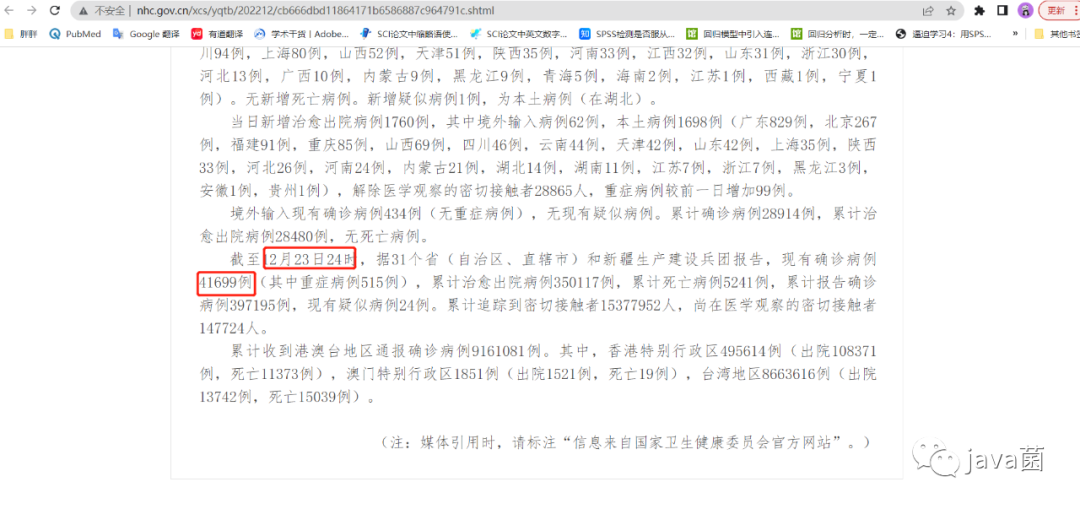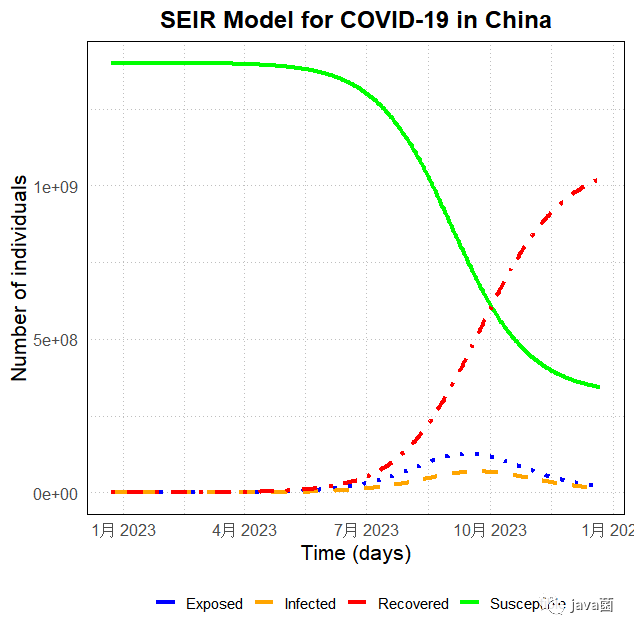+关注继续查看

# 一、引言# 二、seirs简介

「SEIRS模型」是一种流行病学模型，用于「建模呼吸道传染病的传播」。在SEIRS模型中，人口根据其在传染病生命周期中的感染状态被划分为四个类别：「易感（susceptible）、暴露（exposed）、感染（infectious）和康复（recovered）」。SEIRS模型通过考虑个体的人群动态和传染病传播的基本机制，对传染病疫情的演化进行建模。下面是该模型各个状态的定义及其数学表示：

• 「易感状态（S）」：未感染病毒的人群，可以被感染。
• 「暴露状态（E）」：感染病毒但还未出现症状的人群。在这个状态下，人群处于潜伏期，也称为隐藏状态。
• 「感染状态（I）」：患病且具有传染性的人群。
• 「康复状态（R）」：已从疾病中康复的人群，对后续的疾病传播没有贡献。

• 「易感状态」：易感人群可以转变为暴露状态，通过感染的机会是易感人群所接触到已确诊感染的人群。
• 「暴露状态」：暴露人群可以转变为感染状态。根据COVID-19的传播方式，一般潜伏期在9天左右，在这期间，暴露人群会变得有传染性，这也是模型中一个重要的变量。
• 「感染状态」：感染人群可以转变为康复状态，也可以由于治愈失败而转变为死亡状态。它们所感染的人群一般是其他易感人群，传染性与病毒传染的强度有很大关系。 有一部分从疾病中恢复的人群变成康复状态，对此我将其称为R群体。这个状态表示患病者从病毒中恢复，具备免疫力。
• 「康复状态」：康复状态的人群一般来说可以认为会对病毒具有抵御力，因此在后续的传染爆发阶段中可以不作为传染源。

SEIRS模型可以用以下一系列微分方程来表示：

dS/dt= -beta * S * I / N
dE/dt= beta * S * I / N - sigma * E
dI/dt= sigma * E - gamma * I
dR/dt= gamma * I

# 三、数据来源install.packages("deSolve")
library(deSolve)
N <- 1400000000 # 中国总人口数量
beta <- 0.13 # 病毒传播速率
gamma <- 0.995 # 治愈率（包括确诊与无症状感染者）
sigma <- 1/14 # 平均潜伏期
I <- 41699 #初始感染人数

# 四、处理方法

library(deSolve)
# 定义 SEIRS 模型
seirs <- function(time, state, parms) {
with(as.list(c(state, parms)), {
# 模型方程
dS <-  -beta*S*I/(S+E+I+R)
dE <- beta*S*I/(S+E+I+R) - rho*E
dI <- rho*E - gamma*I
dR <- gamma*I
# 返回微分方程组
return(list(c(dS, dE, dI, dR)))
})
}
# 设置模型参数
parms <- c(S = 1.4e9, E = 1, I = 41699, R = 0, rho = 1/14, gamma = 0.13, beta = 0.25)
# 设置初始状态
init <- c(S = 1.4e9, E = 1, I = 41699, R = 0)
# 设置起始时间为今天
start_time <- as.Date("2022-12-23")
# 模拟未来 N 天的趋势
N <- 365
times <- seq(start_time, by = "day", length.out = N)
times <- times <- as.numeric(times)
out <- as.data.frame(ode(y = init, times = times, func = seirs, parms = parms))
# 可视化结果
library(ggplot2)
df <- data.frame(time=as.Date(out$time, origin = "1970-01-01") , S=out[,2], E=out[,3], I=out[,4], R=out[,5]) # 美化图表及设置线条属性 theme_set(theme_minimal(base_size = 14)) # 设置主题 line_size <- 1.5 # 设置线的宽度 line_type <- c('solid', 'dotted', 'dashed', 'dotdash') # 设置不同种类线条的样式 # 绘制图表 ggplot(df, aes(x = time)) + geom_line(aes(y = S, color = "Susceptible"), size = line_size, linetype = line_type) + geom_line(aes(y = E, color = "Exposed"), size = line_size, linetype = line_type) + geom_line(aes(y = I, color = "Infected"), size = line_size, linetype = line_type) + geom_line(aes(y = R, color = "Recovered"), size = line_size, linetype = line_type) + scale_color_manual(values = c("blue", "orange", "red", "green")) + # 自定义颜色 labs(title = "SEIR Model for COVID-19 in China", x = "Time (days)", y = "Number of individuals", color = "Cases") + theme(legend.position = "bottom", legend.title = element_blank(), axis.title = element_text(size = 16), axis.text = element_text(size = 12), plot.title = element_text(size = 18, face = "bold", hjust = 0.5), panel.border = element_rect(colour = "black", fill = NA), panel.grid.major = element_line(colour = "gray", linetype = "dotted", size = 0.5), panel.grid.minor = element_line(colour = "gray", linetype = "dotted", size = 0.3)) # 输出峰值时间和数量 peak_time <- df$time[which.max(df$I)] peak_count <- max(df$I)
cat(paste("Peak time: ", peak_time, " days"))
cat("\n")
cat(paste("Peak count: ", peak_count, " individuals"))

Peak time:  2023-09-22
Peak count:  69027262.4873488# 五、结果解释

「在使用 SEIRS 模型预测传染病的传播时，正确地设置初始参数十分重要。例如，初始感染人数、初始接触人数等参数可能会对结果产生很大的影响。因此，需要对模型进行敏感性分析，确定最优的初始参数。」|
27天前
|

r语言机器学习逻辑回归代码实战 结果分析和折线图
r语言机器学习逻辑回归代码实战 结果分析和折线图
14 1
|
3月前
|

R语言之数值型描述分析
R语言之数值型描述分析
26 0
|
4月前
|

142 0
|
4月前
|

R语言中如何进行PCA分析？利用ggplot和prcomp绘制基因表达量分析图（下）
R语言中如何进行PCA分析？利用ggplot和prcomp绘制基因表达量分析图（下）
55 0
|
4月前
R语言中如何进行PCA分析？利用ggplot和prcomp绘制基因表达量分析图（上）
R语言中如何进行PCA分析？利用ggplot和prcomp绘制基因表达量分析图
57 0
|
4月前
|

143 0
|
4月前
|

46 0
|
4月前
|

COVID-19是当前全球面临的一项重大挑战。 本文将介绍如何使用R语言分析COVID-19相关数据，探索其感染率、死亡率和人口特征的相关性，以及使用统计建模方法预测COVID-19的死亡率。
41 0
|
6月前
|

47 0
|
6月前
|

91 0